Essential Ideas

# 5 Measurements

### Learning Objectives

By the end of this section, you will be able to:

• Explain the process of measurement
• Identify the three basic parts of a quantity
• Describe the properties and units of length, mass, volume, density, temperature, and time
• Perform basic unit calculations and conversions in the metric and other unit systems

Measurements provide much of the information that informs the hypotheses, theories, and laws describing the behavior of matter and energy in both the macroscopic and microscopic domains of chemistry. Every measurement provides three kinds of information: the size or magnitude of the measurement (a number); a standard of comparison for the measurement (a unit); and an indication of the uncertainty of the measurement. While the number and unit are explicitly represented when a quantity is written, the uncertainty is an aspect of the measurement result that is more implicitly represented and will be discussed later.

The number in the measurement can be represented in different ways, including decimal form and scientific notation. (Scientific notation is also known as exponential notation; a review of this topic can be found in Appendix B.) For example, the maximum takeoff weight of a Boeing 777-200ER airliner is 298,000 kilograms, which can also be written as 2.98105 kg. The mass of the average mosquito is about 0.0000025 kilograms, which can be written as 2.510−6 kg.

Units, such as liters, pounds, and centimeters, are standards of comparison for measurements. A 2-liter bottle of a soft drink contains a volume of beverage that is twice that of the accepted volume of 1 liter. The meat used to prepare a 0.25-pound hamburger weighs one-fourth as much as the accepted weight of 1 pound. Without units, a number can be meaningless, confusing, or possibly life threatening. Suppose a doctor prescribes phenobarbital to control a patient’s seizures and states a dosage of “100” without specifying units. Not only will this be confusing to the medical professional giving the dose, but the consequences can be dire: 100 mg given three times per day can be effective as an anticonvulsant, but a single dose of 100 g is more than 10 times the lethal amount.

The measurement units for seven fundamental properties (“base units”) are listed in (Figure). The standards for these units are fixed by international agreement, and they are called the International System of Units or SI Units (from the French, Le Système International d’Unités). SI units have been used by the United States National Institute of Standards and Technology (NIST) since 1964. Units for other properties may be derived from these seven base units.

Base Units of the SI System
Property Measured Name of Unit Symbol of Unit
length meter m
mass kilogram kg
time second s
temperature kelvin K
electric current ampere A
amount of substance mole mol
luminous intensity candela cd

Everyday measurement units are often defined as fractions or multiples of other units. Milk is commonly packaged in containers of 1 gallon (4 quarts), 1 quart (0.25 gallon), and one pint (0.5 quart). This same approach is used with SI units, but these fractions or multiples are always powers of 10. Fractional or multiple SI units are named using a prefix and the name of the base unit. For example, a length of 1000 meters is also called a kilometer because the prefix kilo means “one thousand,” which in scientific notation is 103 (1 kilometer = 1000 m = 103 m). The prefixes used and the powers to which 10 are raised are listed in (Figure).

Common Unit Prefixes
Prefix Symbol Factor Example
femto f 10−15 1 femtosecond (fs) = 110−15 s (0.000000000000001 s)
pico p 10−12 1 picometer (pm) = 110−12 m (0.000000000001 m)
nano n 10−9 4 nanograms (ng) = 410−9 g (0.000000004 g)
micro µ 10−6 1 microliter (μL) = 110−6 L (0.000001 L)
milli m 10−3 2 millimoles (mmol) = 210−3 mol (0.002 mol)
centi c 10−2 7 centimeters (cm) = 710−2 m (0.07 m)
deci d 10−1 1 deciliter (dL) = 110−1 L (0.1 L )
kilo k 103 1 kilometer (km) = 1103 m (1000 m)
mega M 106 3 megahertz (MHz) = 3106 Hz (3,000,000 Hz)
giga G 109 8 gigayears (Gyr) = 8109 yr (8,000,000,000 yr)
tera T 1012 5 terawatts (TW) = 51012 W (5,000,000,000,000 W)

### SI Base Units

The initial units of the metric system, which eventually evolved into the SI system, were established in France during the French Revolution. The original standards for the meter and the kilogram were adopted there in 1799 and eventually by other countries. This section introduces four of the SI base units commonly used in chemistry. Other SI units will be introduced in subsequent chapters.

#### Length

The standard unit of length in both the SI and original metric systems is the meter (m). A meter was originally specified as 1/10,000,000 of the distance from the North Pole to the equator. It is now defined as the distance light in a vacuum travels in 1/299,792,458 of a second. A meter is about 3 inches longer than a yard ((Figure)); one meter is about 39.37 inches or 1.094 yards. Longer distances are often reported in kilometers (1 km = 1000 m = 103 m), whereas shorter distances can be reported in centimeters (1 cm = 0.01 m = 10−2 m) or millimeters (1 mm = 0.001 m = 10−3 m).

The relative lengths of 1 m, 1 yd, 1 cm, and 1 in. are shown (not actual size), as well as comparisons of 2.54 cm and 1 in., and of 1 m and 1.094 yd.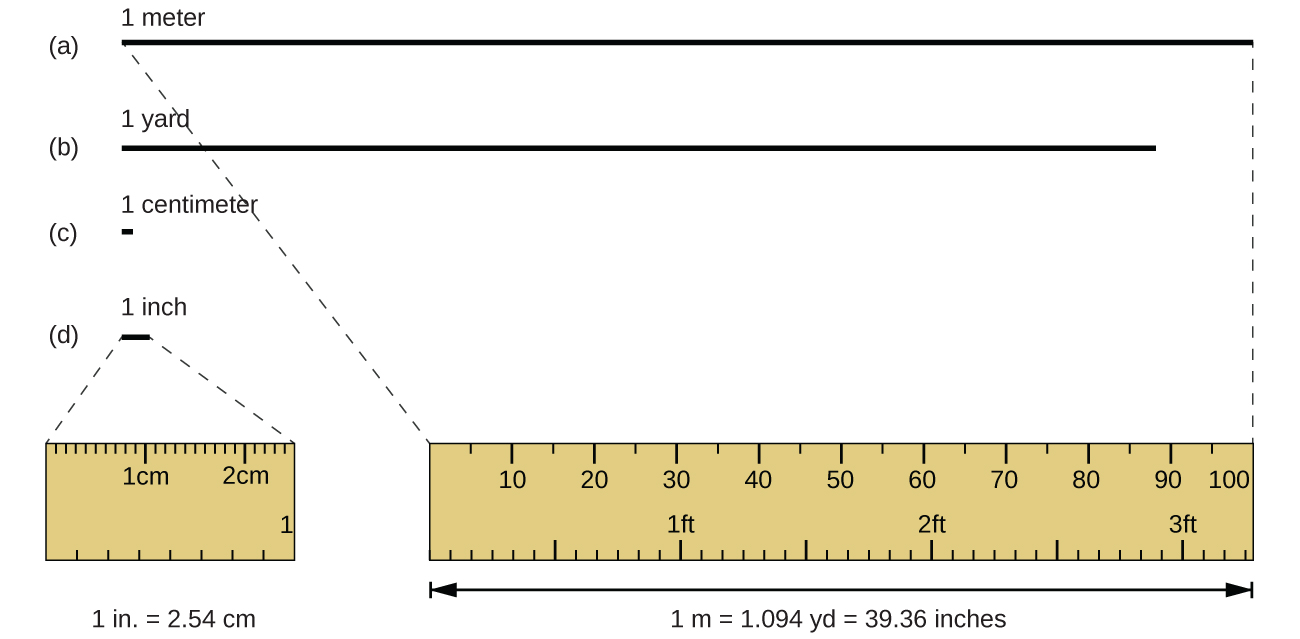#### Mass

The standard unit of mass in the SI system is the kilogram (kg). The kilogram was previously defined by the International Union of Pure and Applied Chemistry (IUPAC) as the mass of a specific reference object. This object was originally one liter of pure water, and more recently it was a metal cylinder made from a platinum-iridium alloy with a height and diameter of 39 mm ((Figure)). In May 2019, this definition was changed to one that is based instead on precisely measured values of several fundamental physical constants.1. One kilogram is about 2.2 pounds. The gram (g) is exactly equal to 1/1000 of the mass of the kilogram (10−3 kg).

This replica prototype kilogram as previously defined is housed at the National Institute of Standards and Technology (NIST) in Maryland. (credit: National Institutes of Standards and Technology)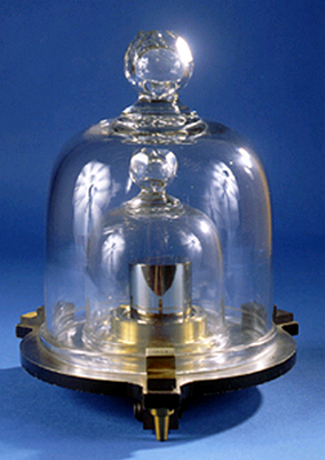#### Temperature

Temperature is an intensive property. The SI unit of temperature is the kelvin (K). The IUPAC convention is to use kelvin (all lowercase) for the word, K (uppercase) for the unit symbol, and neither the word “degree” nor the degree symbol (°). The degree Celsius (°C) is also allowed in the SI system, with both the word “degree” and the degree symbol used for Celsius measurements. Celsius degrees are the same magnitude as those of kelvin, but the two scales place their zeros in different places. Water freezes at 273.15 K (0 °C) and boils at 373.15 K (100 °C) by definition, and normal human body temperature is approximately 310 K (37 °C). The conversion between these two units and the Fahrenheit scale will be discussed later in this chapter.

#### Time

The SI base unit of time is the second (s). Small and large time intervals can be expressed with the appropriate prefixes; for example, 3 microseconds = 0.000003 s = 310−6 and 5 megaseconds = 5,000,000 s = 5106 s. Alternatively, hours, days, and years can be used.

### Derived SI Units

We can derive many units from the seven SI base units. For example, we can use the base unit of length to define a unit of volume, and the base units of mass and length to define a unit of density.

#### Volume

Volume is the measure of the amount of space occupied by an object. The standard SI unit of volume is defined by the base unit of length ((Figure)). The standard volume is a cubic meter (m3), a cube with an edge length of exactly one meter. To dispense a cubic meter of water, we could build a cubic box with edge lengths of exactly one meter. This box would hold a cubic meter of water or any other substance.

A more commonly used unit of volume is derived from the decimeter (0.1 m, or 10 cm). A cube with edge lengths of exactly one decimeter contains a volume of one cubic decimeter (dm3). A liter (L) is the more common name for the cubic decimeter. One liter is about 1.06 quarts.

A cubic centimeter (cm3) is the volume of a cube with an edge length of exactly one centimeter. The abbreviation cc (for cubic centimeter) is often used by health professionals. A cubic centimeter is equivalent to a milliliter (mL) and is 1/1000 of a liter.

(a) The relative volumes are shown for cubes of 1 m3, 1 dm3 (1 L), and 1 cm3 (1 mL) (not to scale). (b) The diameter of a dime is compared relative to the edge length of a 1-cm3 (1-mL) cube.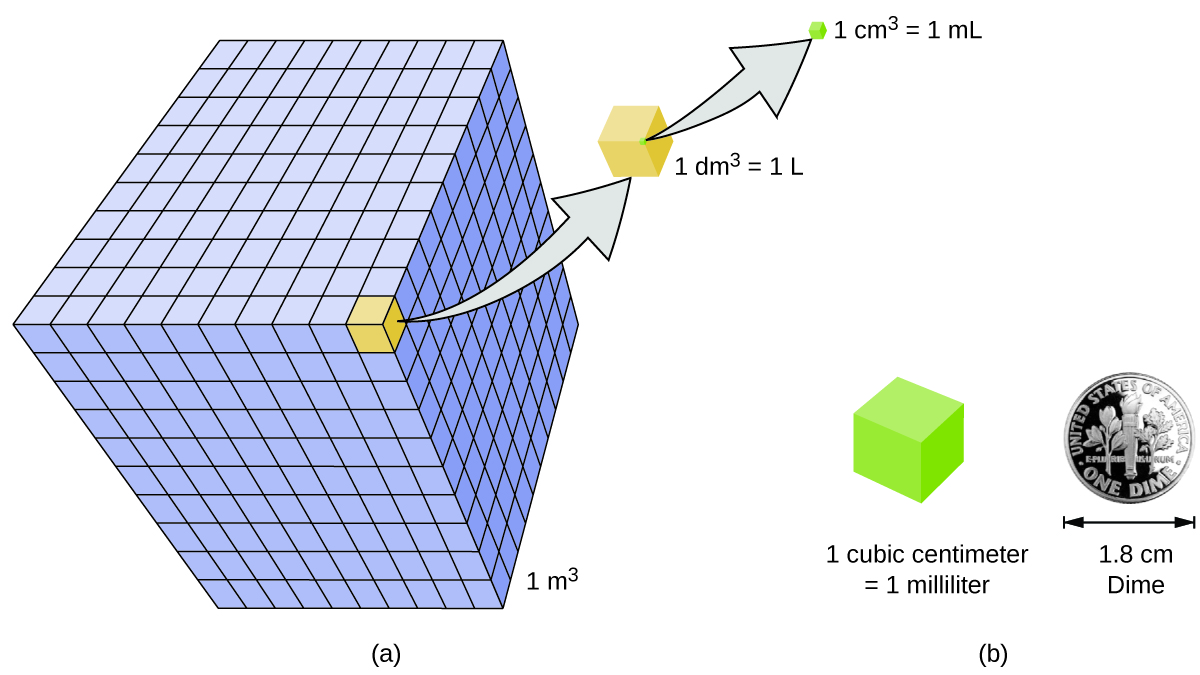#### Density

We use the mass and volume of a substance to determine its density. Thus, the units of density are defined by the base units of mass and length.

The density of a substance is the ratio of the mass of a sample of the substance to its volume. The SI unit for density is the kilogram per cubic meter (kg/m3). For many situations, however, this as an inconvenient unit, and we often use grams per cubic centimeter (g/cm3) for the densities of solids and liquids, and grams per liter (g/L) for gases. Although there are exceptions, most liquids and solids have densities that range from about 0.7 g/cm3 (the density of gasoline) to 19 g/cm3 (the density of gold). The density of air is about 1.2 g/L. (Figure) shows the densities of some common substances.

Densities of Common Substances
Solids Liquids Gases (at 25 °C and 1 atm)
ice (at 0 °C) 0.92 g/cm3 water 1.0 g/cm3 dry air 1.20 g/L
oak (wood) 0.60–0.90 g/cm3 ethanol 0.79 g/cm3 oxygen 1.31 g/L
iron 7.9 g/cm3 acetone 0.79 g/cm3 nitrogen 1.14 g/L
copper 9.0 g/cm3 glycerin 1.26 g/cm3 carbon dioxide 1.80 g/L
lead 11.3 g/cm3 olive oil 0.92 g/cm3 helium 0.16 g/L
silver 10.5 g/cm3 gasoline 0.70–0.77 g/cm3 neon 0.83 g/L
gold 19.3 g/cm3 mercury 13.6 g/cm3 radon 9.1 g/L

While there are many ways to determine the density of an object, perhaps the most straightforward method involves separately finding the mass and volume of the object, and then dividing the mass of the sample by its volume. In the following example, the mass is found directly by weighing, but the volume is found indirectly through length measurements.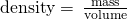Calculation of Density Gold—in bricks, bars, and coins—has been a form of currency for centuries. In order to swindle people into paying for a brick of gold without actually investing in a brick of gold, people have considered filling the centers of hollow gold bricks with lead to fool buyers into thinking that the entire brick is gold. It does not work: Lead is a dense substance, but its density is not as great as that of gold, 19.3 g/cm3. What is the density of lead if a cube of lead has an edge length of 2.00 cm and a mass of 90.7 g?

Solution The density of a substance can be calculated by dividing its mass by its volume. The volume of a cube is calculated by cubing the edge length.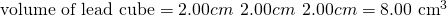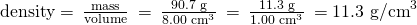(We will discuss the reason for rounding to the first decimal place in the next section.)

Check Your Learning (a) To three decimal places, what is the volume of a cube (cm3) with an edge length of 0.843 cm?

(b) If the cube in part (a) is copper and has a mass of 5.34 g, what is the density of copper to two decimal places?

Answer:

(a) 0.599 cm3; (b) 8.91 g/cm3

Using Displacement of Water to Determine Density This PhET simulation illustrates another way to determine density, using displacement of water. Determine the density of the red and yellow blocks.

Solution When you open the density simulation and select Same Mass, you can choose from several 5.00-kg colored blocks that you can drop into a tank containing 100.00 L water. The yellow block floats (it is less dense than water), and the water level rises to 105.00 L. While floating, the yellow block displaces 5.00 L water, an amount equal to the weight of the block. The red block sinks (it is more dense than water, which has density = 1.00 kg/L), and the water level rises to 101.25 L.

The red block therefore displaces 1.25 L water, an amount equal to the volume of the block. The density of the red block is: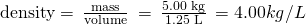Note that since the yellow block is not completely submerged, you cannot determine its density from this information. But if you hold the yellow block on the bottom of the tank, the water level rises to 110.00 L, which means that it now displaces 10.00 L water, and its density can be found: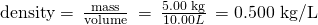Check Your Learning Remove all of the blocks from the water and add the green block to the tank of water, placing it approximately in the middle of the tank. Determine the density of the green block.

Answer:

2.00 kg/L

### Key Concepts and Summary

Measurements provide quantitative information that is critical in studying and practicing chemistry. Each measurement has an amount, a unit for comparison, and an uncertainty. Measurements can be represented in either decimal or scientific notation. Scientists primarily use SI (International System) units such as meters, seconds, and kilograms, as well as derived units, such as liters (for volume) and g/cm3 (for density). In many cases, it is convenient to use prefixes that yield fractional and multiple units, such as microseconds (10−6 seconds) and megahertz (106 hertz), respectively.

### Key Equations

•### Chemistry End of Chapter Exercises

Is one liter about an ounce, a pint, a quart, or a gallon?

Is a meter about an inch, a foot, a yard, or a mile?

about a yard

Indicate the SI base units or derived units that are appropriate for the following measurements:

(a) the length of a marathon race (26 miles 385 yards)

(b) the mass of an automobile

(c) the volume of a swimming pool

(d) the speed of an airplane

(e) the density of gold

(f) the area of a football field

(g) the maximum temperature at the South Pole on April 1, 1913

Indicate the SI base units or derived units that are appropriate for the following measurements:

(a) the mass of the moon

(b) the distance from Dallas to Oklahoma City

(c) the speed of sound

(d) the density of air

(e) the temperature at which alcohol boils

(f) the area of the state of Delaware

(g) the volume of a flu shot or a measles vaccination

(a) kilograms; (b) meters; (c) kilometers/second; (d) kilograms/cubic meter; (e) kelvin; (f) square meters; (g) cubic meters

Give the name and symbol of the prefixes used with SI units to indicate multiplication by the following exact quantities.

(a) 103

(b) 10−2

(c) 0.1

(d) 10−3

(e) 1,000,000

(f) 0.000001

Give the name of the prefix and the quantity indicated by the following symbols that are used with SI base units.

(a) c

(b) d

(c) G

(d) k

(e) m

(f) n

(g) p

(h) T

(a) centi-,10−2; (b) deci-,10−1; (c) Giga-,109; (d) kilo-,103; (e) milli-,10−3; (f) nano-,10−9; (g) pico-,10−12; (h) tera-,1012

A large piece of jewelry has a mass of 132.6 g. A graduated cylinder initially contains 48.6 mL water. When the jewelry is submerged in the graduated cylinder, the total volume increases to 61.2 mL.

(a) Determine the density of this piece of jewelry.

(b) Assuming that the jewelry is made from only one substance, what substance is it likely to be? Explain.

Visit this PhET density simulation and select the Same Volume Blocks.

(a) What are the mass, volume, and density of the yellow block?

(b) What are the mass, volume and density of the red block?

(c) List the block colors in order from smallest to largest mass.

(d) List the block colors in order from lowest to highest density.

(e) How are mass and density related for blocks of the same volume?

(a) 8.00 kg, 5.00 L, 1.60 kg/L; (b) 2.00 kg, 5.00 L, 0.400 kg/L; (c) red < green < blue < yellow; (d) If the volumes are the same, then the density is directly proportional to the mass.

Visit this PhET density simulation and select Custom Blocks and then My Block.

(a) Enter mass and volume values for the block such that the mass in kg is less than the volume in L. What does the block do? Why? Is this always the case when mass < volume?

(b) Enter mass and volume values for the block such that the mass in kg is more than the volume in L. What does the block do? Why? Is this always the case when mass > volume?

(c) How would (a) and (b) be different if the liquid in the tank were ethanol instead of water?

(d) How would (a) and (b) be different if the liquid in the tank were mercury instead of water?

Visit this PhET density simulation and select Mystery Blocks.

(a) Pick one of the Mystery Blocks and determine its mass, volume, density, and its likely identity.

(b) Pick a different Mystery Block and determine its mass, volume, density, and its likely identity.

(c) Order the Mystery Blocks from least dense to most dense. Explain.

(a) (b) Answer is one of the following. A/yellow: mass = 65.14 kg, volume = 3.38 L, density = 19.3 kg/L, likely identity = gold. B/blue: mass = 0.64 kg, volume = 1.00 L, density = 0.64 kg/L, likely identity = apple. C/green: mass = 4.08 kg, volume = 5.83 L, density = 0.700 kg/L, likely identity = gasoline. D/red: mass = 3.10 kg, volume = 3.38 L, density = 0.920 kg/L, likely identity = ice; and E/purple: mass = 3.53 kg, volume = 1.00 L, density = 3.53 kg/L, likely identity = diamond. (c) B/blue/apple (0.64 kg/L) < C/green/gasoline (0.700 kg/L) < D/red/ice (0.920 kg/L) < E/purple/diamond (3.53 kg/L) < A/yellow/gold (19.3 kg/L)

### Footnotes

• 1For details see https://www.nist.gov/pml/weights-and-measures/si-units-mass

### Glossary

Celsius (°C)
unit of temperature; water freezes at 0 °C and boils at 100 °C on this scale
cubic centimeter (cm3 or cc)
volume of a cube with an edge length of exactly 1 cm
cubic meter (m3)
SI unit of volume
density
ratio of mass to volume for a substance or object
kelvin (K)
SI unit of temperature; 273.15 K = 0 ºC
kilogram (kg)
standard SI unit of mass; 1 kg = approximately 2.2 pounds
length
measure of one dimension of an object
liter (L)
(also, cubic decimeter) unit of volume; 1 L = 1,000 cm3
meter (m)
standard metric and SI unit of length; 1 m = approximately 1.094 yards
milliliter (mL)
1/1,000 of a liter; equal to 1 cm3
second (s)
SI unit of time
SI units (International System of Units)
standards fixed by international agreement in the International System of Units (Le Système International d’Unités)
unit
standard of comparison for measurements
volume
amount of space occupied by an object

## LicenseChemistry 2e by OSCRiceUniversity is licensed under a Creative Commons Attribution 4.0 International License, except where otherwise noted.